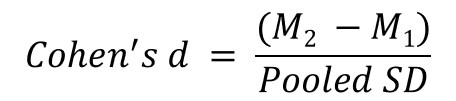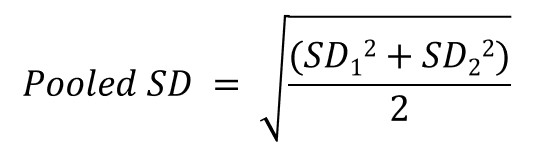Cohen’s d Calculator: A Quick And Easy Method

Below is the Cohen’s d calculator. Simply enter the groups mean and standard deviation values into the calculator, click the calculate button and Cohen’s d values will be created for you.

For further information about the Cohen’s d formula and how it works, we have written an article which covers this in detail. Check it out by clicking here.

How the calculator works

The formula for the Cohen’s d calculator can be seen below. Briefly, it is the mean difference between two groups, divided by the pooled standard deviation (SD).Where the pooled SD is calculated as: